# [Unity3D]Unity3D游戏开发之自由视角下的角色控制

各位朋友，大家好，我是秦元培，欢迎大家关注我的博客，我的博客地址是blog.csdn.net/qinyuanpei。在上一篇文章<[Unity3D]Unity3D游戏开发之角色控制漫谈>一文中，博主与大家分享自己在角色控制方面的一些感悟。今天呢，我们继续来探讨Unity3D角色控制的内容，今天博主将解决在上一篇文章中没有解决的问题，即自由视角下的角色控制。如图是博主非常喜欢的《古剑奇谭》游戏截图，在这款游戏中就使用了博主今天要讲解的自由视角，所谓自由视角是指玩家可以按照自身坐标系向着四个不同的方向移动，当玩家按下鼠标右键时，可以绕Y轴按照一定的角度旋转摄像机，在旋转的过程中，角色将旋转相应的角度。在移动的过程中，摄像机会保持与玩家间的一定距离，然后跟随角色进行移动。好了，下面我们正式开始今天的内容吧！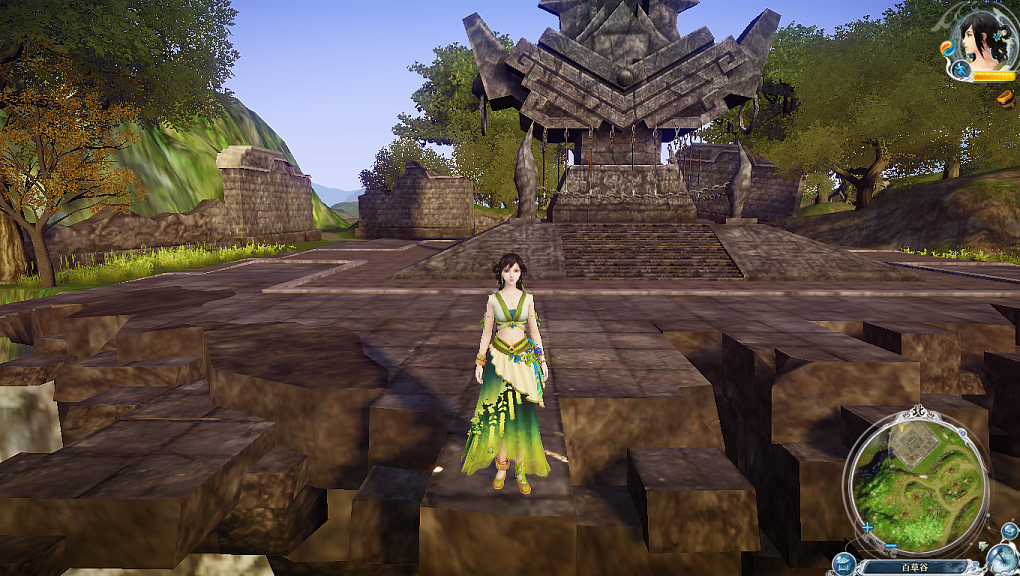在开始今天的内容前，首先让我们来学习下Unity3D中较为重要的一部分知识，理解这些知识是我们开始学习今天内容的基础。

1、Input.GetAxis():该方法用于在Unity3D中根据坐标轴名称返回虚拟坐标系中的值，通常情况下，使用控制器和键盘输入时此值范围在-1到1之间。这段话怎么理解呢？我们来看下面这段脚本：

using UnityEngine;
using System.Collections;

public class example : MonoBehaviour {

//水平速度
public float HorizontalSpeed = 2.0F;
//垂直速度
public float VerticalSpeed = 2.0F;

void Update()
{
//水平方向
float h = HorizontalSpeed * Input.GetAxis("Mouse X");
//垂直方向
float v = VerticalSpeed * Input.GetAxis("Mouse Y");
//旋转
transform.Rotate(v, h, 0);
}
}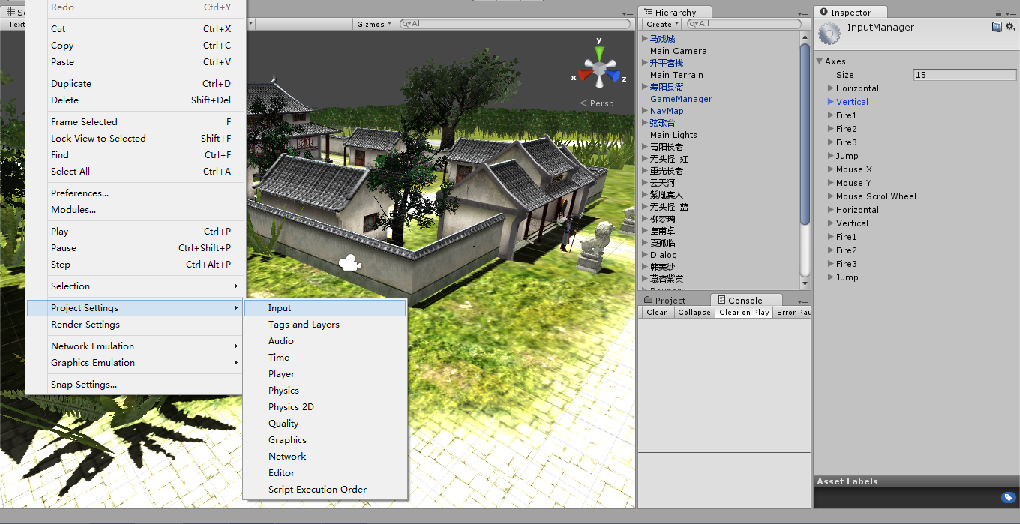2、欧拉角eulerAngles：该值是Vector3类型的值，x、y、z分别代表绕x轴旋转x度，绕y轴旋转y度，绕z轴旋转z度。因此，该值最为直观的形式是可以允许我们直接以一个三维向量的形式来修改一个物体的角度，例如下面的脚本：

float mY = 5.0;

void Update ()
{
mY += Input.GetAxis("Horizontal");
transform.eulerAngles =new Vector3(0,mY, 0);
}
如果你已经理解了上面的话，那么不出意外的，这段脚本会如你所愿的，按照鼠标在水平方向上移动的方向绕Y轴旋转。通常情况下，我们不会单独设置欧拉角其中一个轴，例如eulerAngles.x = 10，因为这将导致偏移和不希望的旋转。当设置它们一个新的值时，要同时设置全部。好在我们可以通过Quaternion.Euler()方法将一个Vector3类型的值转化为一个四元数，进而通过修改Transform.Rotation来实现相同的目的。

3、插值：所谓插值是指离散数据的基础上补插连续函数，使得这条连续曲线通过全部给定的离散数据点。插值是离散函数逼近的重要方法，利用它可通过函数在有限个点处的取值状况，估算出函数在其他点处的近似值。在某些情况下，如果我们希望过程中处理得较为平滑，此时我们就可以使用插值的方法来实现对中间过程的模拟。在Unity3D中我们可以使用两种插值方法，即线性插值Lerp，球形插值SLerp。我们来看下面的脚本：

void Rotating (float horizontal, float vertical)
{
// Create a new vector of the horizontal and vertical inputs.
Vector3 targetDirection = new Vector3(horizontal, 0f, vertical);

// Create a rotation based on this new vector assuming that up is the global y axis.
Quaternion targetRotation = Quaternion.LookRotation(targetDirection, Vector3.up);

// Create a rotation that is an increment closer to the target rotation from the player's rotation.
Quaternion newRotation = Quaternion.Lerp(rigidbody.rotation, targetRotation, turnSmoothing * Time.deltaTime);

// Change the players rotation to this new rotation.
rigidbody.MoveRotation(newRotation);
}


好了，有了这三部分的基础，我们就可以开始今天的内容了，今天的脚本分为两个部分，第一部分是角色控制的部分，主要负责的角色在场景中的移动、转身和动画处理。第二部分是相机控制的部分，主要涉及相机旋转、相机缩放的相关内容。下面，我们分别来讲这两个部分，场景依然是博主自己在做的小游戏：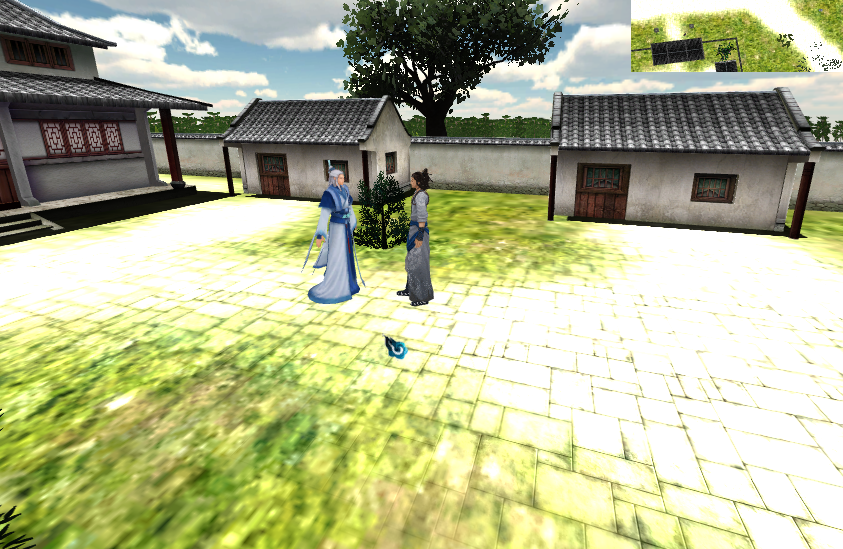using UnityEngine;
using System.Collections;

public class NoLockiVew_Player : MonoBehaviour {

/*自由视角下的角色控制*/
/*作者：秦元培*/

//玩家的行走速度
public float WalkSpeed=1.5F;
//重力
public float Gravity=20;

//角色控制器
private CharacterController mController;
//动画组件
private Animation mAnim;
//玩家方向，默认向前
private DirectionType mType=DirectionType.Direction_Forward;

[HideInInspector]
//玩家状态，默认为Idle
public PlayerState State=PlayerState.Idle;

//定义玩家的状态枚举
public enum PlayerState
{
Idle,
Walk
}

//定义四个方向的枚举值，按照逆时针方向计算
protected enum DirectionType
{
Direction_Forward=90,
Direction_Backward=270,
Direction_Left=180,
Direction_Right=0
}

void Start ()
{
//获取角色控制器
mController=GetComponent<CharacterController>();
//获取动画组件
mAnim=GetComponentInChildren<Animation>();
}

void Update ()
{
MoveManager();
//MouseEvent();
}

//玩家移动控制
void MoveManager()
{
//移动方向
Vector3 mDir=Vector3.zero;
if(mController.isGrounded)
{
//将角色旋转到对应的方向
if(Input.GetAxis("Vertical")==1)
{
SetDirection(DirectionType.Direction_Forward);
mDir=Vector3.forward * Time.deltaTime * WalkSpeed;
State=PlayerState.Walk;
}
if(Input.GetAxis("Vertical")==-1)
{
SetDirection(DirectionType.Direction_Backward);
mDir=Vector3.forward * Time.deltaTime * WalkSpeed;
State=PlayerState.Walk;
}
if(Input.GetAxis("Horizontal")==-1)
{
SetDirection(DirectionType.Direction_Left);
mDir=Vector3.forward * Time.deltaTime * WalkSpeed;
State=PlayerState.Walk;
}
if(Input.GetAxis("Horizontal")==1)
{
SetDirection(DirectionType.Direction_Right);
mDir=Vector3.forward * Time.deltaTime * WalkSpeed;
State=PlayerState.Walk;
}
//角色的Idle动画
if(Input.GetAxis("Vertical")==0 && Input.GetAxis("Horizontal")==0)
{
State=PlayerState.Idle;
}

}
//考虑重力因素
mDir=transform.TransformDirection(mDir);
float y=mDir.y-Gravity *Time.deltaTime;
mDir=new Vector3(mDir.x,y,mDir.z);
mController.Move(mDir);
}

//设置角色的方向，有问题
void SetDirection(DirectionType mDir)
{
if(mType!=mDir)
{
transform.Rotate(Vector3.up*(mType-mDir));
mType=mDir;
}
}
}


using UnityEngine;
using System.Collections;

public class NoLockView_Camera : MonoBehaviour
{
//观察目标
public Transform Target;
//观察距离
public float Distance = 5F;
//旋转速度
private float SpeedX=240;
private float SpeedY=120;
//角度限制
private float  MinLimitY = 5;
private float  MaxLimitY = 180;

//旋转角度
private float mX = 0.0F;
private float mY = 0.0F;

//鼠标缩放距离最值
private float MaxDistance=10;
private float MinDistance=1.5F;
//鼠标缩放速率
private float ZoomSpeed=2F;

//是否启用差值
public bool isNeedDamping=true;
//速度
public float Damping=2.5F;

void Start ()
{
//初始化旋转角度
mX=transform.eulerAngles.x;
mY=transform.eulerAngles.y;
}

void LateUpdate ()
{
//鼠标右键旋转
if(Target!=null && Input.GetMouseButton(1))
{
//获取鼠标输入
mX += Input.GetAxis("Mouse X") * SpeedX * 0.02F;
mY -= Input.GetAxis("Mouse Y") * SpeedY * 0.02F;
//范围限制
mY = ClampAngle(mY,MinLimitY,MaxLimitY);
}

//鼠标滚轮缩放

Distance-=Input.GetAxis("Mouse ScrollWheel") * ZoomSpeed;
Distance=Mathf.Clamp(Distance,MinDistance,MaxDistance);

//重新计算位置和角度
Quaternion mRotation = Quaternion.Euler(mY, mX, 0);
Vector3 mPosition = mRotation * new Vector3(0.0F, 0.0F, -Distance) + Target.position;

//设置相机的角度和位置
if(isNeedDamping){
//球形插值
transform.rotation = Quaternion.Lerp(transform.rotation,mRotation, Time.deltaTime*Damping);
//线性插值
transform.position = Vector3.Lerp(transform.position,mPosition, Time.deltaTime*Damping);
}else{
transform.rotation = mRotation;
transform.position = mPosition;
}
//将玩家转到和相机对应的位置上
if(Target.GetComponent<NoLockiVew_Player>().State==NoLockiVew_Player.PlayerState.Walk)
{
Target.eulerAngles=new Vector3(0,mX,0);
}
}

private float  ClampAngle (float angle,float min,float max)
{
if (angle < -360) angle += 360;
if (angle >  360) angle -= 360;
return Mathf.Clamp (angle, min, max);
}

}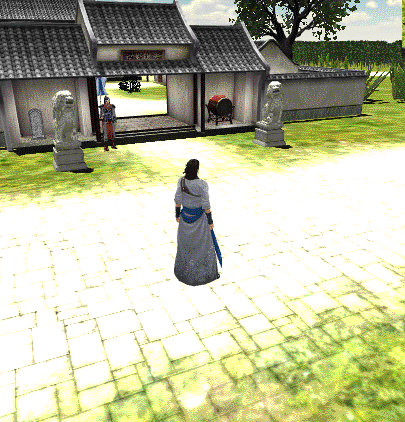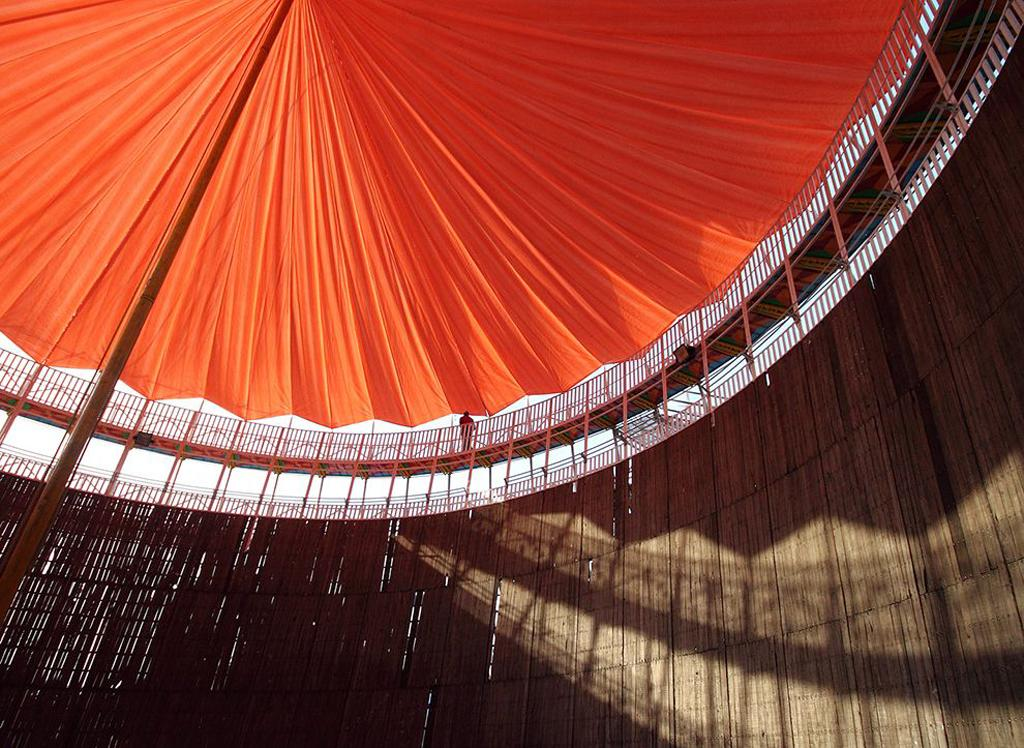喜欢我的博客请记住我的名字：秦元培，我博客地址是blog.csdn.net/qinyuanpei。
转载请注明出处，本文作者：秦元培，本文出处：http://blog.csdn.net/qinyuanpei/article/details/39125353

文章更新：

9月10日，博主在测试这个项目的时候意外地发现了一个Bug。Bug出现在如下位置：

		//设置玩家跟随角度
if(Target.GetComponent<NoLockiVew_Player>().State==NoLockiVew_Player.PlayerState.Walk)
{
Target.rotation=Quaternion.Euler(new Vector3(0,mX,0));
}

using UnityEngine;
using System.Collections;

public class NoLockView_Camera : MonoBehaviour
{
//观察目标
public Transform Target;
//观察距离
public float Distance = 5F;
//旋转速度
private float SpeedX=240;
private float SpeedY=120;
//角度限制
private float  MinLimitY = 5;
private float  MaxLimitY = 180;

//旋转角度
private float mX = 0.0F;
private float mY = 0.0F;

//鼠标缩放距离最值
private float MaxDistance=10;
private float MinDistance=1.5F;
//鼠标缩放速率
private float ZoomSpeed=2F;

//是否启用差值
public bool isNeedDamping=true;
//速度
public float Damping=10F;

private Quaternion mRotation;

void Start ()
{
//初始化旋转角度
mX=transform.eulerAngles.x;
mY=transform.eulerAngles.y;
}

void LateUpdate ()
{
//鼠标右键旋转
if(Target!=null && Input.GetMouseButton(1))
{
//获取鼠标输入
mX += Input.GetAxis("Mouse X") * SpeedX * 0.02F;
mY -= Input.GetAxis("Mouse Y") * SpeedY * 0.02F;
//范围限制
mY = ClampAngle(mY,MinLimitY,MaxLimitY);
//计算旋转
mRotation = Quaternion.Euler(mY, mX, 0);
//根据是否插值采取不同的角度计算方式
if(isNeedDamping){
transform.rotation = Quaternion.Lerp(transform.rotation,mRotation, Time.deltaTime*Damping);
}else{
transform.rotation = mRotation;
}
//处理同时按下鼠标右键和方向控制键
if(Target.GetComponent<NoLockiVew_Player>().State==NoLockiVew_Player.PlayerState.Walk){
Target.rotation=Quaternion.Euler(new Vector3(0,mX,0));
}
}

//鼠标滚轮缩放
Distance-=Input.GetAxis("Mouse ScrollWheel") * ZoomSpeed;
Distance=Mathf.Clamp(Distance,MinDistance,MaxDistance);

//重新计算位置
Vector3 mPosition = mRotation * new Vector3(0.0F, 0.0F, -Distance) + Target.position;

//设置相机的角度和位置
if(isNeedDamping){
transform.position = Vector3.Lerp(transform.position,mPosition, Time.deltaTime*Damping);
}else{
transform.position = mPosition;
}

}

//角度限制
private float  ClampAngle (float angle,float min,float max)
{
if (angle < -360) angle += 360;
if (angle >  360) angle -= 360;
return Mathf.Clamp (angle, min, max);
}
}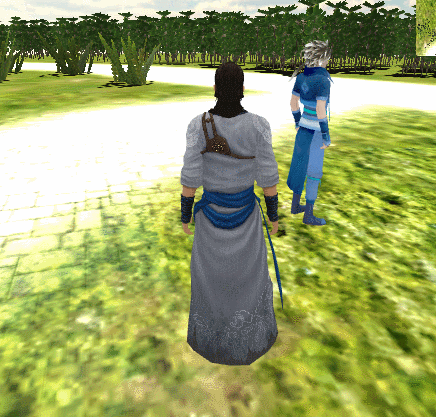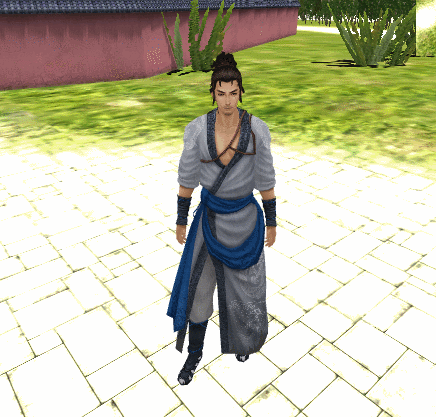03-25
11-30387105-121万+
09-15
09-095263
07-04
04-22